# 生成模型

## 0.生成模型介绍1、Component-by-Component

2、AutoEncoder

## 1.Component-by-Component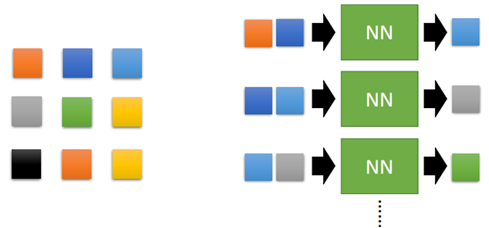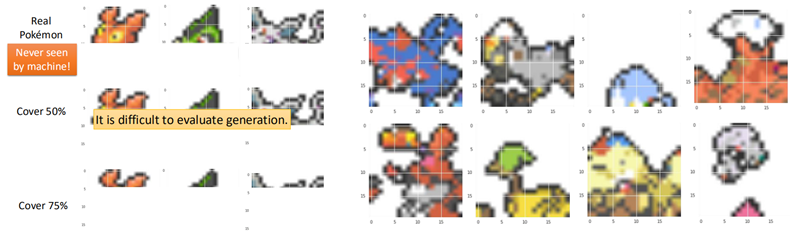## 2.AutoEncoder

### 2.1 Review AutoEncoder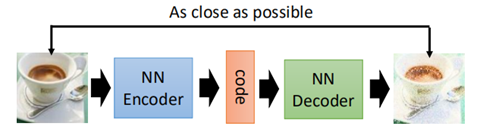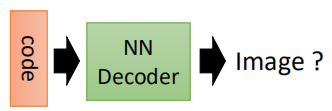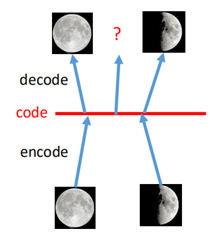### 2.2 VAE简介

VAE在进行图片还原时，要保证code与decoder的输出服从一定的分布，所以V的意思代表Variational。换句话说，就是在生成code的时候我们限制这些code服从一定的分布

VAE的直观理解是，在生成的code上加上一些“噪音”，如图：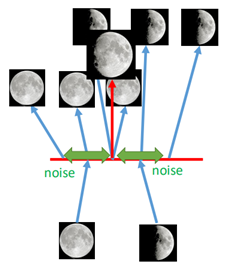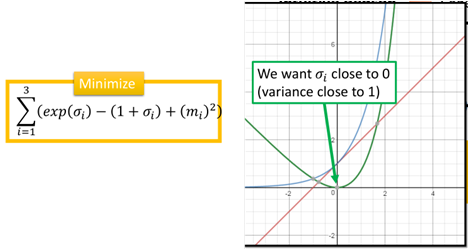exp(σ)为蓝色的线，（1+σ）为红色的线，二者相减则为绿色的线，最小化这一项，则使得σi在0附近，再取exp，那么varaice则趋向于1，最后一项m的平方则可以看做是L2正则化。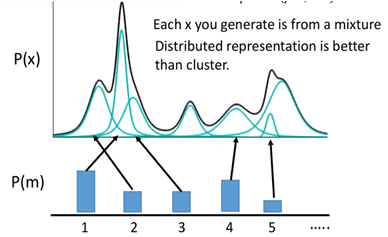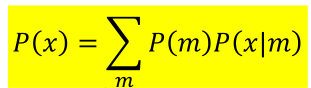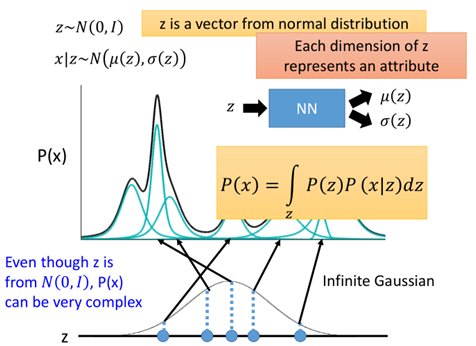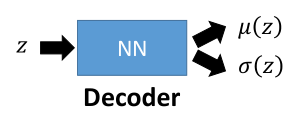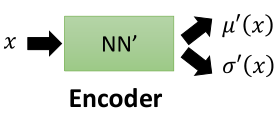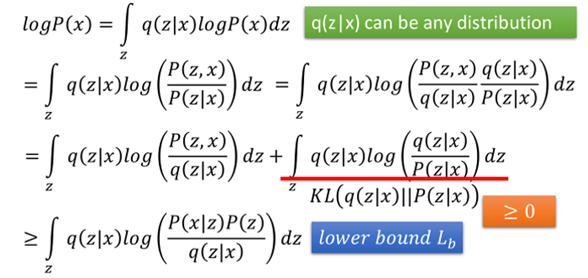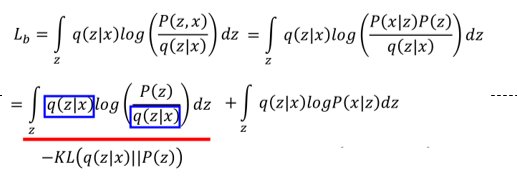### 2.3 VAE的问题

VAE虽然能够比较容易地产生一些数据，但其实VAE并没有学会如何真正的去生成一些新的事物，而是一直在模仿，希望尽可能地接近已知样本。比如：对于生成的两张图片“7”，第一张显然对于我们来说是可以接受的，而第二种是不可接受的，然而对于VAE来说，二者具有相同的损失（重构误差）。同时通过实验可以看出，VAE生成的图片一般比较“糊”，这是因为autoencoder在生成图片时，每一个pixel是独立的，它并没有考虑相互之间的关系（大局观）。

这时就需要另一个生成模型登场了——GAN。

### 2.4 VAE的实现

``````import tensorflow as tf
import numpy as np
import matplotlib.pyplot as plt
from tensorflow.examples.tutorials.mnist import input_data

# 读取数据

# 输入占位符，因为是无监督学习，所以只需要x
x = tf.placeholder(tf.float32, [None, 784])

# 定义变量从输入层到隐藏层的w,b，隐藏层假设有100个节点
encode_w = tf.Variable(tf.truncated_normal([784, 100], stddev=0.01))
encode_b = tf.Variable(tf.zeros())

# 定义隐藏层到输出m那一层的权重w，假设降维到128维
encode_mean_w = tf.Variable(tf.truncated_normal([100, 128], stddev=0.01))
# 定义隐藏层到输出的variance的权重
encode_var_w = tf.Variable(tf.truncated_normal([100, 128], stddev=0.01))

# code到输出层的w和b
decode_w = tf.Variable(tf.truncated_normal([128, 784], stddev=0.01))
decode_b = tf.Variable(tf.zeros())

# 隐藏层输出
encode_output = tf.nn.relu(tf.matmul(x, encode_w) + encode_b)
# 求解mean和variance
encode_mean = tf.matmul(encode_output, encode_mean_w)
encode_var = tf.matmul(encode_output, encode_var_w)
# 加上一个random normal
E = tf.random_normal([1, 128])
# 降维后的数据 m + exp(var) * E

# decoder,把code解回原数据784维
decode_output = tf.nn.relu(tf.matmul(code, decode_w) + decode_b)

# loss，原来的重构误差
decode_loss = tf.reduce_mean((decode_output - x) ** 2)
# 加上另一项误差
encode_loss = tf.reduce_mean(tf.exp(encode_var) - (1 + encode_var) + encode_mean ** 2)

# tf.reset_default_graph()

with tf.Session() as sess:
sess.run(tf.global_variables_initializer())
for epoch in range(10000):
# total_num = int(mnist.train.num_examples/100)
# for i in range(total_num):
xs, ys = mnist.train.next_batch(100)
_, loss_ = sess.run([optimizer, loss], feed_dict={x: xs})

if epoch % 100 == 0:
print('epoch:', epoch, 'loss:', loss_)

# test
I_test = tf.truncated_normal(shape=[1, 128], stddev=0.00001)

decode_output_test = tf.nn.relu(tf.matmul(I_test, decode_w) + decode_b)

decode_output_test_data = sess.run([decode_output_test])

test_img = np.reshape(decode_output_test_data, [28, 28])

plt.imshow(test_img, cmap='gray')

plt.pause(0.1)``````

##``````
# 这里首先定义一个max_pool函数，返回的经过max_pool之后的图片和所对应的索引
def max_pool_with_argmax(net, stride):
_, mask = tf.nn.max_pool_with_argmax(net, ksize=[1, stride, stride, 1], strides=[1, stride, stride, 1], padding='SAME')
net = tf.nn.max_pool(net, ksize=[1, stride, stride, 1], strides=[1, stride, stride, 1], padding='SAME')

# 根据max_pool的索引进行反池化的操作，原理在前面CNN部分已经说过
ksize = [1, stride, stride, 1]
input_shape = net.get_shape().as_list()

output_shape = (input_shape, input_shape * ksize, input_shape * ksize, input_shape)

batch_range = tf.reshape(tf.range(output_shape, dtype=tf.int64), shape=[input_shape, 1, 1, 1])
y = mask // (output_shape * output_shape)
x = mask % (output_shape * output_shape) // output_shape
feature_range = tf.range(output_shape, dtype=tf.int64)

indices = tf.transpose(tf.reshape(tf.stack([b, y, x, f]), [4, updates_size]))
ret = tf.scatter_nd(indices, values, output_shape)
return ret

x = tf.placeholder(tf.float32, [100, 28, 28, 1])

w_conv1 = tf.Variable(tf.truncated_normal([3, 3, 1, 64], stddev=0.01))
b_conv1 = tf.constant(0.1, shape=)

conv1 = tf.nn.relu(tf.nn.conv2d(x, w_conv1, strides=[1, 1, 1, 1], padding='SAME') + b_conv1)

w_conv2 = tf.Variable(tf.truncated_normal([3, 3, 64, 10], stddev=0.01))
b_conv2 = tf.constant(0.1, shape=)

conv2 = tf.nn.relu(tf.nn.conv2d(pool1, w_conv2, strides=[1, 1, 1, 1], padding='SAME') + b_conv2)
# pool2 = tf.nn.max_pool2d(conv2, ksize=[1, 3, 3, 1], strides=[1, 3, 3, 1], padding='SAME')

conv_out = tf.reshape(pool2, [-1, 490])

encode_w = tf.Variable(tf.truncated_normal([490, 100]))
encode_b = tf.Variable(tf.constant(0.1, shape=))

encode_mean_w = tf.Variable(tf.truncated_normal([100, 128], stddev=0.01))
encode_var_w = tf.Variable(tf.truncated_normal([100, 128], stddev=0.01))

encode_mean = tf.matmul(encode_output, encode_mean_w)
encode_var = tf.matmul(encode_output, encode_var_w)

E = tf.random_normal([1, 128])

code = tf.add(tf.exp(encode_var) * E, encode_mean)

# decoder

decode_w = tf.Variable(tf.truncated_normal([128, 490], stddev=0.01))
decode_b = tf.Variable(tf.constant(0.1, shape=))

decode_output = tf.reshape(decode_output, [-1, 7, 7, 10])

t_pool1 = tf.nn.conv2d_transpose(t_conv2 - b_conv2, w_conv2, pool1.shape, [1, 1, 1, 1])

pre_output = tf.nn.conv2d_transpose(t_conv1-b_conv1, w_conv1, x.shape, [1, 1, 1, 1])

decode_loss = tf.reduce_mean((pre_output - x) ** 2)

encode_loss = tf.reduce_mean(tf.exp(encode_var) - (1 + encode_var) + encode_mean ** 2)

with tf.Session() as sess:
sess.run(tf.global_variables_initializer())
for epoch in range(10000):
# total_num = int(mnist.train.num_examples/100)
# for i in range(total_num):
xs, ys = mnist.train.next_batch(100)
xs = np.reshape(xs, [-1, 28, 28, 1])
_, loss_ = sess.run([optimizer, loss], feed_dict={x: xs})

if epoch % 100 == 0:
print('epoch:', epoch, 'loss:', loss_)

# test
I_test = tf.truncated_normal(shape=[1, 128], stddev=0.01)

decode_output_test = tf.reshape(decode_output_test, [-1, 7, 7, 10])

t_pool1_test = tf.nn.conv2d_transpose(t_conv2_test - b_conv2, w_conv2, [1, 14, 14, 64], [1, 1, 1, 1])

pre_output_test = tf.nn.conv2d_transpose(t_conv1_test - b_conv1, w_conv1, [1, 28, 28, 1], [1, 1, 1, 1])

decode_output_test_data = sess.run([pre_output_test], feed_dict={x: xs})

test_img = np.reshape(decode_output_test_data, [28, 28])

plt.imshow(test_img, cmap='gray')

plt.pause(0.1)``````

## 3. GAN简介

GAN全名叫做生成对抗网络，顾名思义，就是在不断地生成和对抗中进行成长学习。举一个例子：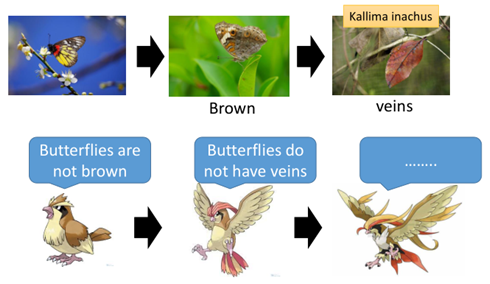图中是枯叶蝶，其天敌是一种鸟类，在最开始时，枯叶蝶可能就是普通的蝴蝶，而这种鸟靠捕食蝴蝶为食，这种鸟认为蝴蝶不是棕色的，因此，蝴蝶进化成棕色的骗过第一代的鸟，而鸟类也在进化，进化成第二代，可以辨别蝴蝶是没有叶脉的，因此蝴蝶进一步进化成枯叶蝶。这其实就是一种对抗生成。

那么在实际的机器学中，对抗生成网络有两个部分组成，一个是Generator，另一个是Discriminator，二者在不断进行生成与对抗，称为亦师亦友的关系。在图片生成中：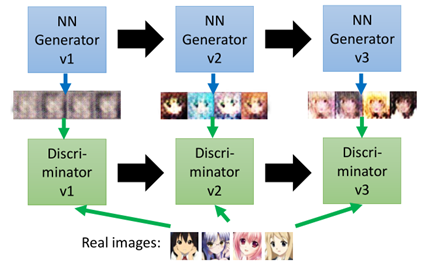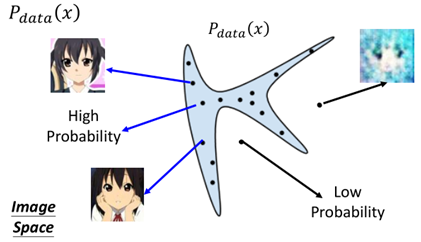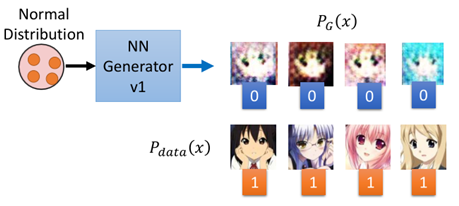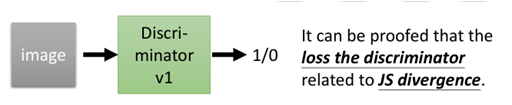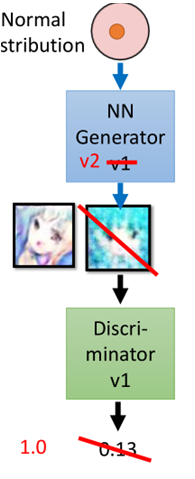“””

Generator：G，Discriminator:  D

• Initialize G θg, D θd(初始化Generator和Discriminator的参数)
• for each training
• sample m examples{x1, x2, …..,xm} from datanse;(从样本集中sample出一些数据)
• sample m noise samples {z1, z2, …..,zm} from a distribution;(从一种分布中sample出一些数据)
• Obtaining generated data  {x’1,x’2,……x’m},x’i=G(zi);（然后把z丢进G中产生一些图片）
• Fix G, update θd to maximize ， （固定住Generator的参数，调整Discriminator的参数，这里并不一定使用这种方法更新，还有其他方法也就衍生了其他算法）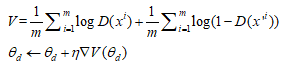• sample m noise samples{z1,z2,……,zm} from a distribution;(再另外从某种分布中sample出一些数据)
• Fix D, update to maximize；（固定住Discriminator，调整Generator的参数，使得sample出来的那些数据让Discriminator的分数越高越好）“””